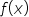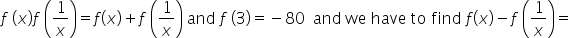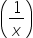Maths-
General
Easy

###Hint:

In this question, we haveis a polynomial function such that. Firstly, we will use the given information to find the value of f(x) and f. Then, we will multiply the equations derived. And later assume g(x) to be f(x)-1 and gto be f-1. Further, we will get the value of f(x) and fand thus we can find the required sum.

## Book A Free Demo+91